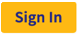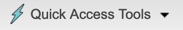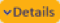Always login to Rguroo through the Rguroo main website: To sign-in to Rguroo, use thebutton on Rguroo’s Home webpage (https://www.rguroo.com). This ensures that you get the latest version of Rguroo, and the Rguroo application gets loaded into your browser correctly.

In addition to routine bug fixes, the following are major additions to the Version 1.7.2:

The dropdownwas added to the top-left of the Rguroo’s application main page to facilitate access to frequently used functions such as Data Import Dataset, Import RGR file, Scientific Calculator, and Shortcut Keys.

### Applets-Calculators Toolbox

An Applets-Calculator toolbox was added. It currently consists of the following applets:

• Rossman/Chance Applet Collection: These applets can be used for teaching statistical concepts, and include the following sections: Sampling Distribution, Data Analysis, Probability, and Statistical Inference.

• Desmos Calculators: These include Scientific Calculator, Four Function Calculator, and a Graphical Calculator.

### Analytics Toolbox

#### One Population Proportion Inference

Simulation-based methods have been added for constructing confidence intervals and performing tests of hypotheses for one population proportion.

• Bootstrap confidence intervals have been added with three options of Percentile, Standard Error (SE), and BCa. Dotplot graphs, showing the simulated values and confidence intervals are included in the output.

• Test of hypothesis based on simulation (parametric bootstrap) has been added. By default, a p-value graph is shown in the report. A critical region graph can be added optionally to the report.

• Power analysis using simulation was added.

#### Two Population Proportion Inference

Simulation-based methods have been added to construct confidence intervals and perform tests of hypotheses for the difference of two populations proportions.

• Bootstrap confidence intervals have been added with two options of Percentile, and Standard Error (SE). Dotplot graphs, showing the simulated values and confidence intervals are included in the output.

• Permutation test for test whether two population proportions differ has been added. By default, a p-value graph is shown in the report. A critical region graph can be added optionally to the report.

#### Analysis of Variance (ANOVA)dialog box was added to allow users to choose between Type I, Type II, or Type III sum of squares.

#### Nonparametric ANOVA

•dialog box added to allow users to choose the number of simulations and random number generator seed for simulation-based one-way and two-way ANOVA methods.

• Graphs related to the Kruskal-Wallis and Friedman methods were added.

### Plot Toolbox

#### Dotplot Case Identification

A function is added to thesection of the dotplot that allows you to identify cases on the dot plot using colors, plot characters, or plot character size.

#### Dotplot Proportions and Counts

In the Function dropdown of the Barplot module under the Numerical tab, two options of “Count” and “Proportion” were added. These options apply when one numerical variable is selected, or one numerical and one factor is selected. The option “Counts” gives the frequency barplot for a numerical variable, and the option “proportion” gives the relative frequency barplot for the selected numerical variable. If a factor variable is selected, then the frequencies and relative frequencies will be plotted for each level of the selected factor.

#### Dotplot labeling change

In the Categorical tab of the Dotplot module, labels changed from “Frequency* to”Counts" and from “Relative Frequency* to”Proportions."

#### Scatterplot Jittering

The option of jittering points in the x- and y- directions were added to the scatterplot module. Go to$$\rightarrow$$ Attributes of Scatterplot ... $$\rightarrow$$ Point-Line and specify values in the X-Jitter or Y-Jitter boxes.

#### Digit Separator

An option of Digit Separator was added to the Tick Label section for all plots that have x- and y- axes. If the number of digits for x-axis or y-axis tick labels is larger than three digits, they can be separated by commas or dots in groups of three. The default was changed, so no digit separator is added. To use the digit seperator option, go to thedialog. Then select Title and Axes $$\rightarrow$$ X-Axis $$\rightarrow$$ Tick Then in the Tick Label section select Digit Sep.

### Data Toolbox

#### Transform Module

• The result of the R code can be either a scalar or a vector of any size. In the previous versions, the results were restricted to be a scalar or the same size as the number of rows of the selected dataset. Variables with smaller number of cases are padded with NA’s if Complete Cases Only is not selected.

• Complete Cases Only was added, so the resulting saved dataset will only include the complete cases. All rows with at least one NA will be removed.

#### Merge Module

If no common variable is specified based on which to merge dataset, the two selected datasets will be merged by case numbers (i.e., concatenated horizontally). The dataset with a larger number of cases will be first. No other options will be available in this case. The rows with no data will be filled with NA’s.

#### Video Tutorials

Video tutorials for one-population inference and dotplot were added.